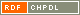# Show document

Title: Vertex-transitive expansions of (1, 3)-trees Lovrečič Saražin, Marko (Author)Marušič, Dragan (Author)http://dx.doi.org/10.1016/j.disc.2009.11.036 English Not categorized 1.08 - Published Scientific Conference Contribution IAM - Andrej Marušič Institute A nonidentity automorphism of a graph is said to be semiregular if all of its orbits are of the same length. Given a graph ▫$X$▫ with a semiregular automorphism ▫$\gamma$▫, the quotient of ▫$X$▫ relative to ▫$\gamma$▫ is the multigraph ▫$X/\gamma$▫ whose vertices are the orbits of ▫$\gamma$▫ and two vertices are adjacent by an edge with multiplicity ▫$r$▫ if every vertex of one orbit is adjacent to ▫$r$▫ vertices of the other orbit. We say that ▫$X$▫ is an expansion of ▫$X/\gamma$▫. In [J.D. Horton, I.Z. Bouwer, Symmetric ▫$Y$▫-graphs and ▫$H$▫-graphs, J. Combin. Theory Ser. B 53 (1991) 114-129], Hortonand Bouwer considered a restricted sort of expansions (which we will call :strong" in this paper) where every leaf of ▫$X/\gamma$▫ expands to a single cycle in ▫$X$▫. They determined all cubic arc-transitive strong expansions of simple ▫$(1,3)$▫-trees, that is, trees with all of their vertice shaving valency 1 or 3, thus extending the classical result of Frucht, Graver and Watkins (see [R. Frucht, J.E. Graver, M.E. Watkins, The groups of the generalized Petersen graphs, Proc. Cambridge Philos. Soc. 70 (1971) 211-218]) about arc-transitive strong expansions of ▫$K_2$▫ (also known as the generalized Petersen graphs). In this paper another step is taken further by considering the possible structure of cubic vertex-transitive expansions of general ▫$(1,3)$▫-multitrees (where vertices with double edges are also allowed); thus the restriction on every leaf to be expanded to a single cycle is dropped. graph, tree, cubic, vertex-transitive, arc-transitive, expansion 2010 str. 1772-1782 Vol. 310, no. 12 0012-365X 519.17 10241923401958 49Document is not linked to any category.

Average score: (0 votes) Voting is allowed only to logged in users.Hover the mouse pointer over a document title to show the abstract or click on the title to get all document metadata.

## Secondary language

Language: Unknown teorija grafov, graf, drevo, kubični grafi, trazitivnost, ekspanzija